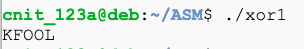ASM 210: XOR (20 pts)

What You Need

• A Google Cloud Debian 10 Linux Server

Purpose

Encrypt and decrypt data using XOR in Assembler.

Understanding XOR

Exclusive OR (XOR) is a fundamental mathematical operation used in many encryption algorithms.

XOR operates on one bit at a time, with these results:

0 XOR 0 = 0
0 XOR 1 = 1
1 XOR 0 = 1
1 XOR 1 = 0
For our purposes, we'll use the Python ^ operator, which acts on a whole byte at a time.

Characters are ASCII-encoded, like this:

A is 01000001
B is 01000010
C is 01000011
...
A whole table of ASCII values is here:

Consider A^B:

A is 01000001
B is 01000010
A^B= 00000011
That is character 3, an unprintable end-of-text mark.

However, A^s is printable:

A is 01000001
s is 01110011
A^B= 00110010
The result is the hexadecimal value 0x32, or the numeral 2.

Simple XOR Program in Assembler

Try this program, which encrypts HELLO with a key of 3:
section  .text
global   _start

_start:

mov  ecx, 5      ; number of characters to encrypt

loop1:
push ecx

mov  ebx, msg    ; start of message
sub  ebx, 1
mov  al, [ebx]   ; address of current character
xor  eax, 3      ; 3 is the key
mov  [ebx], al
pop  ecx
loop loop1

mov  edx, len
mov  ecx, msg
mov  ebx, 1
mov  eax, 4
int  0x80        ; print message

mov  eax, 1
int  0x80        ; exit

section  .data

msg  db  "HELLO", 13, 10
len  equ \$ - msg
Compile and run it. The result is "KFOOL", as shown below.ASM 210.1: XOR with the Key (5 pts)

XOR the text below with a key of 11 to reveal the flag.
XHYJFIGN

ASM 210.2: XOR without the Key (15 pts)

The key is between 1 and 40. XOR the text below to produce a readable flag.

Hint: use procedures.

SZ@[QJ\A

Sources

System calls in the Linux kernel. Part 1.
X86 Assembly/Interfacing with Linux
Linux System Call Table (32-bit)
List of Linux/i386 system calls
Assembly Programming Tutorial

Posted 7-17-2020